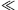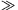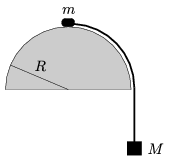Mathematical and Physical Journal
for High Schools
Issued by the MATFUND Foundation
 Already signed up? New to KöMaL?

#Problem P. 4490. (December 2012)

P. 4490. A small body of mass m is placed to the top of a fixed hemisphere, and is tied by means of a rope to another body of mass M, which hangs vertically. The system is released, the small body of mass m slides along the hemisphere without friction. At which mass ratio m/M will the small body of mass m leave the surface of the hemisphere exactly at the angle of 45o? Where will the small body leave the hemisphere if Mm, and where will it leave the surface if Mm?(5 pont)

Deadline expired on January 10, 2013.

Sorry, the solution is available only in Hungarian. Google translation

Megoldásvázlat. Ha $\displaystyle \alpha=45^\circ$, $\displaystyle m/M=7{,}1.$

$\displaystyle m\ll M$ esetén a függőlegestől mért szög $\displaystyle \alpha=25{,}8^\circ$,

$\displaystyle m\gg M$ esetén $\displaystyle \alpha=48{,}2^\circ$.

### Statistics:

 77 students sent a solution. 5 points: Antalicz Balázs, Balogh Menyhért, Barta Szilveszter Marcell, Bingler Arnold, Buttinger Milán, Csáky Pál, Csathó Botond, Csibi Levente, Csóka József, Dávid Bence, Dinev Georgi, Emri Tamás, Fehér Zsombor, Filep Gábor, Forrai Botond, Garai Zoltán, Garami Anna, Holczer András, Janzer Barnabás, Jenei Márk, Juhász Péter, Kaprinai Balázs, Kovács 444 Áron, Majoros Péter, Mezősi Máté, Morvay Bálint, Olosz Balázs, Öreg Botond, Papp Roland, Pázmán Zalán, Sal Kristóf, Sárvári Péter, Seress Dániel, Szabó 928 Attila, Szathmári Balázs, Trócsányi Péter, Vajda Balázs, Váli Tamás, Varju Ákos. 4 points: Blum Balázs, Győrfi 946 Mónika, Kollarics Sándor, Kovács-Deák Máté, Reitz Angéla, Takács 737 Gábor, Ürge László, Wappler Abigél. 3 points: 8 students. 2 points: 12 students. 1 point: 6 students. 0 point: 4 students.

Problems in Physics of KöMaL, December 2012